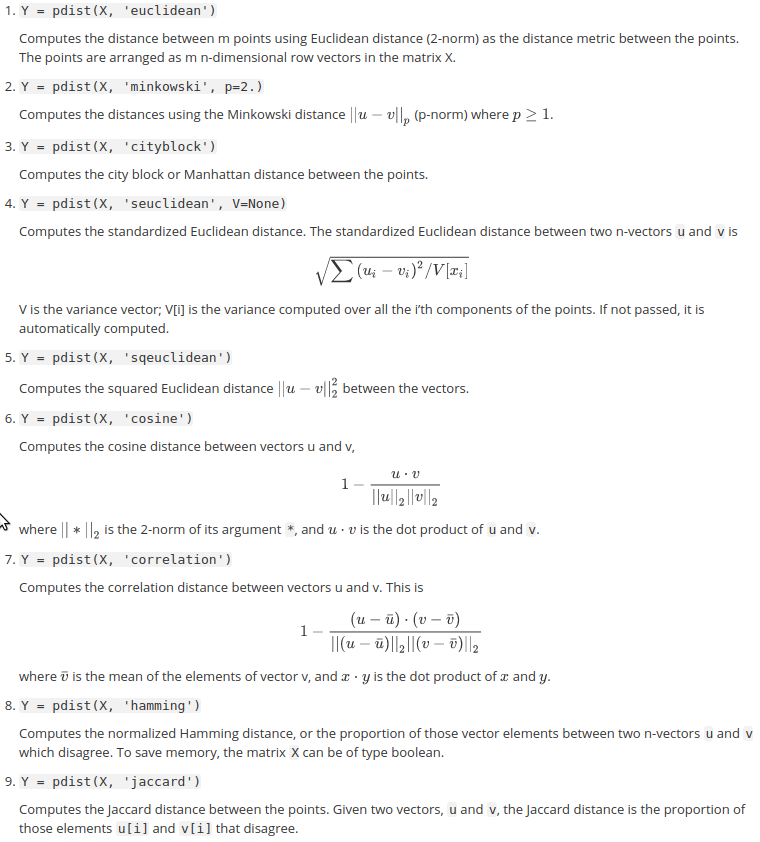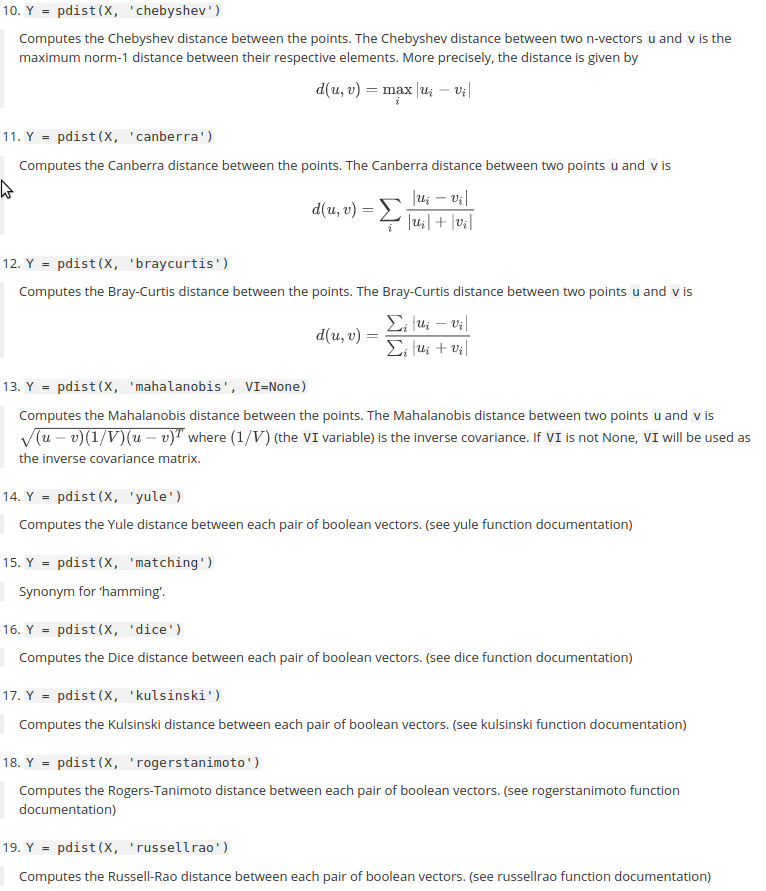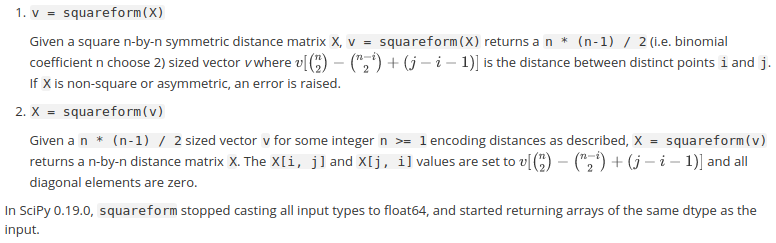scipy.spatial 模块提供了距离计算模块 - scipy.spatial.distance.

Distance computations (scipy.spatial.distance)

## 1. 距离度量函数

### 1.1. 距离函数列表(数值)

1braycurtis(u, v[, w])计算两个 1-D 数组间的 Bray-Curtis distance
2canberra(u, v[, w])计算两个 1-D 数组间的 Canberra distance
3chebyshev(u, v[, w])计算 Chebyshev distance
4cityblock(u, v[, w])计算 City Block (Manhattan) distance
5correlation(u, v[, w, centered]计算 u 和 v 之间的相关系数
6cosine(u, v[, w])计算两个 1-D 数组间的 Cosine distance
7euclidean(u, v[, w])计算两个 1-D 数组间的 Euclidean distance
8jensenshannon(p, q[, base])计算两个 1-D 数组间的 Jensen-Shannon distance (metric)
9mahalanobis(u, v, VI)计算两个 1-D 数组间的 Mahalanobis distance
10minkowski(u, v[, p, w])计算两个 1-D 数组间的 Minkowski distance
11seuclidean(u, v, V)计算两个 1-D 数组间的 standardized Euclidean distance
12sqeuclidean(u, v[, w])计算两个 1-D 数组间的 squared Euclidean distance
13wminkowski(u, v, p, w)计算两个 1-D 数组间的 weighted Minkowski distance

### 1.2. 距离函数列表(二值向量)

1dice(u, v[, w])计算两个 1-D 布尔向量间的 Dice dissimilarity
2hamming(u, v[, w])计算两个 1-D 向量间的 Hamming distance
3jaccard(u, v[, w])计算两个 1-D 布尔向量间的 Jaccard-Needham dissimilarity
4kulsinski(u, v[, w])计算两个 1-D 布尔向量间的 Kulsinski dissimilarity
5rogerstanimoto(u, v[, w])计算两个 1-D 布尔向量间的 Rogers-Tanimoto dissimilarity
6russellrao(u, v[, w])计算两个 1-D 布尔向量间的 Russell-Rao dissimilarity
7sokalmichener(u, v[, w])计算两个 1-D 布尔向量间的 Sokal-Michener dissimilarity
8sokalsneath(u, v[, w])计算两个 1-D 布尔向量间的 Sokal-Sneath dissimilarity
9yule(u, v[, w])计算两个 1-D 布尔向量间的 Yule dissimilarity

### 1.3. cosine

scipy.spatial.distance.cosine(u, v, w=None)
# u - 输入数组
# v - 输入数组
# w - u 和 v 中每个元素值的权重. 默认为 None，每个元素值的权重为 1.0

$$dist = 1 - \frac{u \cdot v}{||u||_2 ||v||_2}$$

from scipy.spatial import distance

distance.cosine([1, 0, 0],
[0, 1, 0])
# 1.0

distance.cosine([100, 0, 0],
[0, 1, 0])
# 1.0

distance.cosine([1, 1, 0],
[0, 1, 0])
# 0.29289321881345254

### 1.4. euclidean

scipy.spatial.distance.euclidean(u, v, w=None)

$$dist = ||u - v||_2 = \sqrt{\sum(w_i|(u_i - v_i)|^2)}$$

from scipy.spatial import distance

distance.euclidean([1, 0, 0],
[0, 1, 0])
# 1.4142135623730951

distance.euclidean([1, 1, 0],
[0, 1, 0])
# 1.0

### 1.5. dice

scipy.spatial.distance.dice(u, v, w=None)

$$dice\_dissimilarity = \frac{c_{TF} + c_{FT}}{2c_{TT} + c_{FT} + c_{TF}}$$

from scipy.spatial import distance

distance.dice([1, 0, 0],
[0, 1, 0])
# 1.0

distance.dice([1, 0, 0],
[1, 1, 0])
# 0.3333333333333333

distance.dice([1, 0, 0],
[2, 0, 0])
#-0.3333333333333333

### 1.6. hamming

$$hamming\_dist = \frac{c_{01} + c_{10}}{n}$$

from scipy.spatial import distance

distance.hamming([1, 0, 0],
[0, 1, 0])
# 0.66666666666666663

distance.hamming([1, 0, 0],
[1, 1, 0])
# 0.33333333333333331

distance.hamming([1, 0, 0],
[2, 0, 0])
# 0.33333333333333331

distance.hamming([1, 0, 0],
[3, 0, 0])
# 0.33333333333333331

## 2. 距离计算函数

scipy.spatial.distance 提供了几种距离计算函数：pdist, cdist, directed_hausdorff.

### 2.1. pdist

doc - scipy.spatial.distance.pdist

Y = scipy.spatial.distance.pdist(X, metric='euclidean', *args, **kwargs)

 - X - n-dim 空间中 m 个观察值组成的 mxn 的数组.

 - metric - 字符串或函数. 距离度量函数.

 - Y - 距离矩阵. 对于每个 i 和 j，其中，i<j<m，m 为观察值数(样本数). 计算 dist(u=X[i], v=X[j]) 度量值，并保存于 Y[ij].

import numpy as np

from scipy.spatial.distance import pdist
from scipy.spatial.distance import squareform

A=np.array([[1,2],
[3,4],
[5,6],
[7,8]])

# A是一个向量矩阵，distA 为数组形式
distA = pdist(A,metric='euclidean')
#array([2.82842712,
#       5.65685425,
#       8.48528137,
#       2.82842712,
#       5.65685425,
#       2.82842712])

# 将 distA 数组变成一个矩阵
distB = squareform(distA)
#array([[0.        , 2.82842712, 5.65685425, 8.48528137],
#       [2.82842712, 0.        , 2.82842712, 5.65685425],
#       [5.65685425, 2.82842712, 0.        , 2.82842712],
#       [8.48528137, 5.65685425, 2.82842712, 0.        ]])### 2.2. cdist

doc - scipy.spatial.distance.cdist

Y = scipy.spatial.distance.cdist(XA, XB, metric='euclidean', *args, **kwargs)

import numpy as np
from scipy.spatial.distance import cdist

A=np.array([[1,2],
[3,4],
[5,6]])
B=np.array([[7,8],
[9,10]])
distAB = cdist(A,B,metric='euclidean')
#array([[ 8.48528137, 11.3137085 ],
#       [ 5.65685425,  8.48528137],
#       [ 2.82842712,  5.65685425]])

### 2.3. squareform

doc - scipy.spatial.distance.squareform

scipy.spatial.distance.squareform(X, force='no', checks=True)Last modification：June 24th, 2020 at 11:21 am